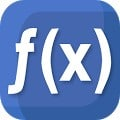# Mathematics APK

– formula calculation with variables, sums, products and sequences
– solve equations (linear, quadratic, cubic, transpose)
– plot functions, derivate, integrate, calculate roots and extremas (polynomial, rational, exponential, etc)
– calculate tangent, asymptote, interception
– reconstruction: calculate f(x) by given roots, points or extremas
– f(x,y) plot in 3D
– factoring (Bézout’s identity, Euler’s totient function, least common multiple, greatest common divisor)
– modulo calculation with big integer and fractions
– differential and integral calculus
– curve sketching, limes, minima, maxima
– linear algebra, vectors, matrices
– unit conversion
– number systems, binary/oct/decimal/hex calculator
– complex number calculation
– statistics (gaussian test, t test, binominal and normal distribution, standard deviation, average, sum, median)Supported languages:
English, German, Francais, Espanol, Italian, Portuguese

Mathematics is a powerful calculation software for your android smartphone.

FEATURES

Calculate any formula you want and show them in a 2d or 3d plot. The natural display shows fractions, roots and exponents as you would expect it from mathematics.

In a few seconds you derivate or integrate your desired function, calculate the zero points of your function und show them in the function plot. See all maxima, minima or inflection points in one view.

The easy way of use allows you to solve linear equations in just a moment. Or transform your mathematical, physical or chemical equation to any unknown variable.

You often needs to calculate with binary, octal or hexadecimal number systems? No problem! You can mix them together in one calculation even by using decimal digits. But that’s not enough! You can also calculate with any other number system with base 2 to 18.

From time to time you may need to convert units to another one, like Celsius to Fahrenheit, miles to kilometre, inches to foot and so on.

You will also be able to calculate with vectors, matrices and determinants.

All this features are combined in this app and will make your mathematical life a lot easier.

#### What’s New

– share your calculations via Bluetooth
– time calculator

Name
Mathematics
Package
de.daboapps.mathematics
Version
3.3
Size
1.91 MB
Installs
Developed By
daboApps

• pashuram chaudhary says:

excellent apps for st.

• sunil says:

Beautiful app!

• computer repair hackney says:

I would have loved to have had this app when I was in school. Although found it to be a little slow.

This site uses Akismet to reduce spam. Learn how your comment data is processed.